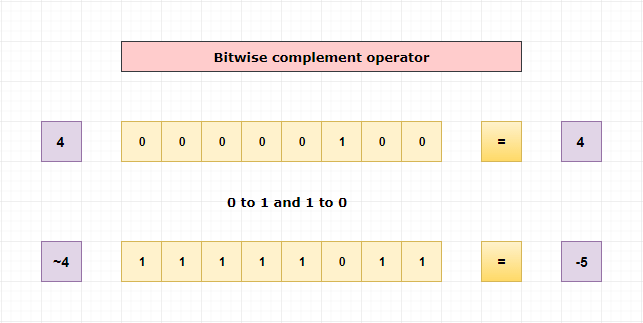# Bitwise one's complement operator (~)

Bitwise one's compliment operator will invert the binary bits.

If a bit is 1, it will change it to 0.

If the bit is 0, it will change it to 1.

#### Example

Let’s take a number 4.

Then ~4 will be,

4 = (00000100) 2

~4 = (11111011) 2.It will convert all 1 to 0 and 0 to 1.

#### Pictorial Explanation## If we print the value of ~4 using printf, it will print -5. How?

Let's take a look on how -5 will be stored in computer?

As we discussed earlier negative numbers are represented using 2’s complement form.

To know how to calculate 2’s complement of a number, kindly refer the link.How integers are stored in memory

So, -5 will be stored like below,

5 = (00000101) 2

1’s complement of 5 = (11111010) 2

Add 1 to get the 2’s complement,

-5 = (11111011) 2

Result of ~4 is equal to the representation of -5 in binary, hence ~4 is -5.

#### Example 2

Let’s take number 2,

2 = (00000010) 2

1’s complement of 2 = (11111101) 2

Add 1 to get the 2’s complement,

~2 = (11111101) 2

Result of ~2 is equal to the representation of -3 in binary, hence ~2 is -3.

## Program using bitwise ones complement operator

Example

```#include<stdio.h>

int main()
{
int var = 3;

printf("value = %d \n",~var); // which is equal to -4

return 0;
}
```